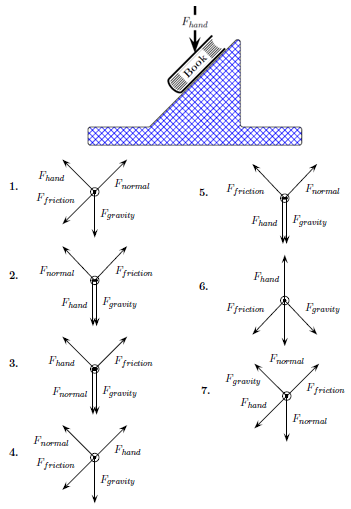Problem: A book is at rest on an incline as shown below. A hand, in contact with the top of the book, produces a constant force Fhand vertically downward. The following figures show several attempts at drawing free-body diagrams for the book. Which figure has the correct directions for each force? The magnitudes of the forces are not necessarily drawn to scale.

FREE Expert Solution
83% (427 ratings)
Problem Details

A book is at rest on an incline as shown below. A hand, in contact with the top of the book, produces a constant force Fhand vertically downward. The following figures show several attempts at drawing free-body diagrams for the book. Which figure has the correct directions for each force? The magnitudes of the forces are not necessarily drawn to scale.